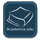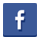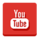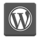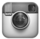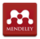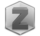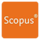You are in:Home/Publications

### Dr. Mohamed Reda Ali Mohamed :: Publications:

Research Fields
 Functions of complex variable Probability and Statistics Partial Differential Equations Modern Algebra Numerical Basic Sciences Mathematics
 Number of publications is 21
 Title 1-The Method of Lines Analysis of Heat Transfer of Ostwald-de Waele Fluid Generated by a Non-uniform Rotating Disk with a Variable Thickness 2-Evolutionary numerical approach for solving nonlinear singular periodic boundary value problems Abstract 3-The method of lines for solution of the carbon nanotubes engine oil nanofluid over an unsteady rotating disk Abstract 4-New exact solutions of Bratu Gelfand model in two dimensions using Lie symmetry analysis Abstract 5-New wavelet method for solving boundary value problems arising from an adiabatic tubular chemical reactor theory Abstract 6-Evolutionary Numerical Approach for Solving Nonlinear Singular Periodic Boundary Value ProblemsAbstract 7-Analytical Solutions for Nonlinear Dispersive Physical Model Abstract 8-Application of a new hybrid method for solving singular fractional Lane–Emden-type equations in astrophysics Abstract 9- A Truncation Method for Solving the Time-Fractional Benjamin-Ono Equation 10-Solution of fractional Volterra-Fredholm integro-differential equations under mixed boundary conditions by using the HOBW method 11-Hybrid Orthonormal Bernstein and Block-Pulse functions wavelet scheme for solving the 2D Bratu problem 12- New Exact Solutions of Nonlinear (3 + 1)-Dimensional Boiti-Leon-Manna-Pempinelli Equation, Advances in Mathematical Physics (2019) 1-8. 13-Solution of nonlinear Volterra integral equations with weakly singular kernel by using the HOBW method 14-Application of Haar wavelet method for solving the nonlinear fuzzy integro-differential equations 15-Numerical Solution of Volterra-Fredholm Integral Equations Using Hybrid Orthonormal Bernstein and Block-Pulse Functions‏ 16-Haar wavelets scheme for solving the unsteady gas flow in four-dimensional 17-Darboux transformation for soliton solutions of the modified Kadomtsev-Petviashvili-II equation 18-Approximate solutions for fuzzy Volterra integrodifferential equations 19-Detection of new multi-wave solutions in an unbounded domain Abstract 20-Numerical Solution of Volterra-Fredholm Integral Equations Using Hybrid Orthonormal Bernstein and Block-Pulse Functions Abstract 21- A comparison between the method of lines and Adomian decomposition method for solving the KdV-Burger equation